Goal Seek: Reverse Engineer An Answer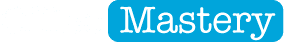Goal Seek: Reverse Engineering For ExcelTrial and error is time consuming. Goal Seek fixes that.

Goal Seek is a simple tool in Excel that lets you go straight to the answer and set it to anything you want. Excel then works backwards and evaluates what input value is required in order for the answer to be achieved.

Feature Download: Grab 2 fantastic takeaways (both free). Watch a free video demonstrating everything discussed below and download a handy cheat sheet (printable reference) summarising all the key steps.

Example 1: Using Goal Seek To Forecast Revenue

Let’s start with a really simple example - a classic sales calculation.

• You sell widgets at \$0.13 each [price].
• A customer orders 2,000 widgets [quantity].
• Your revenue is [price] multiplied by [sales] which gives us \$260.00.

Now, let’s say we want to reach a revenue of \$300.00,

Assuming the same quantity of widgets are ordered, what would we need to increase the price to?

OR … assuming we sell widgets at the same price, what quantity would the customer need to order?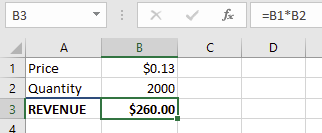Figure 01: Revenue Calculator

Here’s how we would use Goal Seek to calculate the new PRICE.

1.       Select cell B3.

2.      Select Data | What If Analysis | Goal Seek.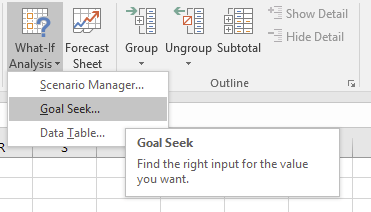Figure 02: Where to find the Goal Seek tool

3.      Change Value to 300.

4.      Set By Changing Cell to B1.Figure 03: A completed Goal Seek dialog

5.       Click OK to see the results.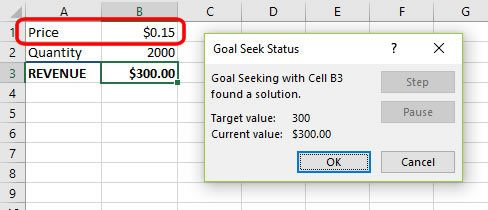Figure 04: The new price projected by Goal Seek

This tells us that we would need to increase the price from \$0.13 to \$0.15 to achieve our revenue target of \$300.00

At this point you can click OK to keep the new price or Cancel to revert back to the original value.

Let’s use Goal Seek again to calculate the new QUANTITY.

1.       Select cell B3.

2.      Select Data | What If Analysis | Goal Seek.

3.      Change ‘To Value’ to 300.

4.      Set ‘By Changing Cell’ to B2.

5.      Click OK.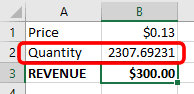Figure 05: The new Quantity projected by Goal Seek

This tells us that we would need to increase the quantity to 2,308 (rounding up the decimals) to achieve our revenue target of \$300.00

Example 2: Using Goal Seek To Calculate Monthly Mortgage Repayments

A mortgage repayment has 3 contributing factors

• The amount borrowed
• The interest rate
• The term (length of the loan)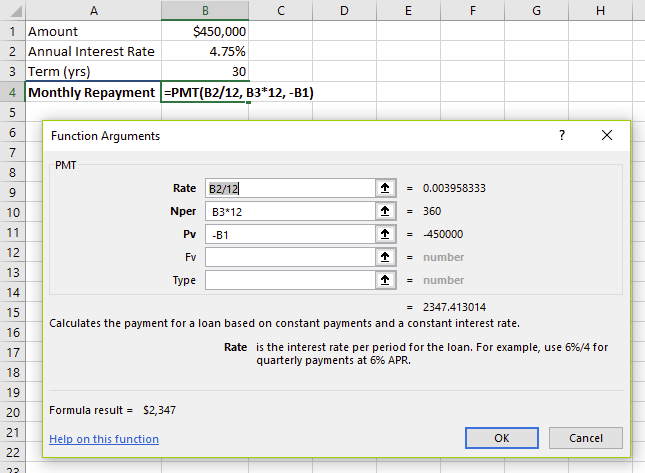Figure 06: The PMT calculation

This is a quick breakdown of the PMT function.

• Rate - annual rate has been divided by 12 to give the MONTHLY rate.
• Term - the number of years (currently 30) has been multiplied by 12 to give the total number of MONTHLY payments
• PV - Present Value (how much are we borrowing?)
• FV - Future Value (e.g. balloon/residual payment at the end of a 3 or 5 year loan). This is optional.
• Type - ‘0’ = Pay at end of month; ‘1’ = pay at beginning of month. This is optional.

Notice how all the figures were adjusted to work on a common MONTHLY basis (i.e. B2/12 and B3*12).

There is a minus sign in front of the Present Value (PV). This makes it negative, which makes the final repayment figure positive.

Anyway back to Goal Seek!

The current monthly repayment based on these figures is \$2,347.

Let’s say you get a pay rise and you can now afford to pay \$2,600 instead of \$2,347.

Using Goal Seek you can discover 3 things:

• Q1  Assuming the same interest rate and term, how much more could you borrow?
• Q2  Assuming the same loan amount and term, what could the interest rate rise to without putting you in danger of not being able to pay?
• Q3  Assuming the same loan amount and interest rate, how much would the term reduce?

Let’s answer those questions.

1.       Select cell B4.

2.      Select Data | What If Analysis | Goal Seek.

3.      Change ‘To Value’ to 2600.

4.      Set ‘By Changing Cell’ to B1.

5.      Click OK.

The amount you can borrow has increased from \$450,000 to \$498,421.

And setting ‘By Changing Cell’ to B2 … The interest rate could rise from 4.75% to 5.66%.

And setting ‘By Changing Cell’ to B3 … The term reduces from 30 years to 24.37 years.

So that’s Goal Seek. A simple tool that provides incredible functionality and purpose.

Enjoy.

Did this clear something up for you? If so, please share on your socials and/or post a brief comment below.

Likewise, if something didn't make sense or you have a question, pop it into the comments below. I'll be waiting ...

Cheers. Here's to your learning and success. Enjoy the rest of your day.FREE CHEAT SHEET & VIDEO

Watch the video to see it done and download a printable PDF cheat sheet of all the key steps

PLUS, GET A FREE COPY OF MY 30 EXCEL POWER TIPS